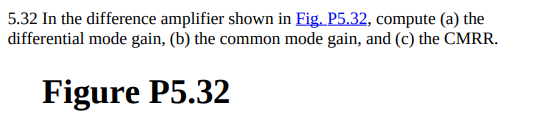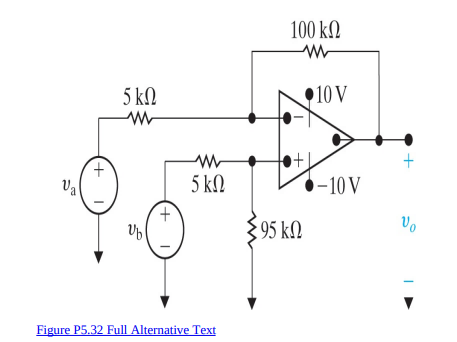# Question 5.32 In the difference amplifier shown in Fig. $$\mathrm{P} 5.32$$, compute (a) the differential mode gain, (b) the common mode gain, and (c) the CMRR. I-19ure P5.32>Figure P5.32 Full Alternative TextSXFGHE The Asker · Other NSM
5.32 In the difference amplifier shown in Fig. $$\mathrm{P} 5.32$$, compute (a) the differential mode gain, (b) the common mode gain, and (c) the CMRR.
I-19ure P5.32>Figure P5.32 Full Alternative TextTranscribed Image Text: 5.32 In the difference amplifier shown in Fig. $$\mathrm{P} 5.32$$, compute (a) the differential mode gain, (b) the common mode gain, and (c) the CMRR. I-19ure P5.32>Figure P5.32 Full Alternative Text
More
Transcribed Image Text: 5.32 In the difference amplifier shown in Fig. $$\mathrm{P} 5.32$$, compute (a) the differential mode gain, (b) the common mode gain, and (c) the CMRR. I-19ure P5.32>Figure P5.32 Full Alternative Text